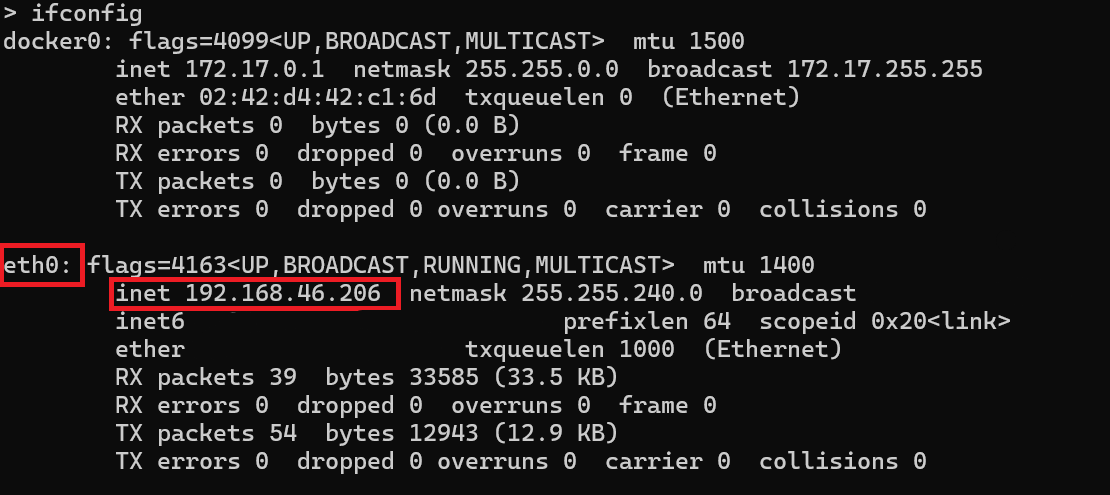Sign Following Robot with ROS 2 in Simulink

Use Simulink® to control a simulated robot running on a separate ROS-based simulator over ROS 2 network.

In this example, you run a model that implements a sign-following algorithm and controls the simulated robot to follow a path based on signs in the environment. The algorithm receives the location information and camera information from the simulated robot, which is running in a separate ROS-based simulator. The algorithm detects the color of the sign and sends the velocity commands to turn the robot based on the color. In this example, the algorithm is designed to turn left when robot encounters a blue sign and turn right when robot encounters a green sign. FInally the robot stops when it encounters a red sign.

To see this example using ROS 1 or MATLAB®, see Sign Following Robot with ROS in MATLAB.

Start Robot Simulator

Start a ROS-based simulator for a differential-drive robot and configure the Simulink® connection with the robot simulator.

This example uses a virtual machine (VM) available for download using instructions in Get Started with Gazebo and a Simulated TurtleBot.

• Start the Ubuntu® virtual machine desktop.

• In the Ubuntu desktop, click the Gazebo ROS2 Maze icon to start the Gazebo world built for this example.

• In MATLAB Command Window, set the ROS_DOMAIN_ID environment variable to 25 to match the robot simulator settings and run ros2 topic list to verify that the topics from the robot simulator are visible in MATLAB.

setenv('ROS_DOMAIN_ID','25')
ros2('topic','list')
/parameter_events

Setup the Simulink ROS preferences to communicate with the robot simulator.

Open the example model.

open_system('signFollowingRobotROS2.slx');

To configure the network settings for ROS 2.

• Under the Simulation tab, in PREPARE, select ROS Toolbox > ROS Network.

• In Configure ROS Network Addresses, set the ROS 2 Domain ID value to 25.

• Click OK to apply changes and close the dialog.

On each time step, the algorithm detects a sign from the camera feed, decides on turn and drives it forward. The sign detection is done in the Image Proecessing subsystem of the model.

open_system('signFollowingRobotROS2/Image Processing');

The Sign Tracking Logic subsystem implements a Stateflow® chart that takes in the detected image size and coordinates from Image Processing and provides linear and angular velocity to drive the robot.

open_system('signFollowingRobotROS2/Sign Tracking Logic');

Run the Model

Run the model and observe the behavior of the robot in the robot simulator.

• The video viewers show the actual camera feed and the detected sign image.

• In the simulator, the robot follows the sign and turns based on the color.

• The simulation stops automatically once the robot reaches the red sign at the end.